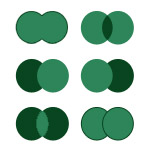Remember my article on Set Operations in the Unix Shell? I implemented 14 various set operations by using common Unix utilities such as diff, comm, head, tail, grep, wc and others. I decided to create a simpler version of that post that just lists the operations. I also created a .txt cheat-sheet version of it and to make things more interesting I added an Awk implementation of each set op. If you want a detailed explanations of each operation, go to the original article.

Download path: `https://catonmat.net/ftp/setops.txt`

## Set Membership

```\$ grep -xc 'element' set    # outputs 1 if element is in set
# outputs >1 if set is a multi-set
# outputs 0 if element is not in set

\$ grep -xq 'element' set    # returns 0 (true)  if element is in set
# returns 1 (false) if element is not in set

\$ awk '\$0 == "element" { s=1; exit } END { exit !s }' set
# returns 0 if element is in set, 1 otherwise.

\$ awk -v e='element' '\$0 == e { s=1; exit } END { exit !s }'
```

## Set Equality

```\$ diff -q <(sort set1) <(sort set2) # returns 0 if set1 is equal to set2
# returns 1 if set1 != set2

\$ diff -q <(sort set1 | uniq) <(sort set2 | uniq)
# collapses multi-sets into sets and does the same as previous

\$ awk '{ if (!(\$0 in a)) c++; a[\$0] } END{ exit !(c==NR/2) }' set1 set2
# returns 0 if set1 == set2
# returns 1 if set1 != set2

\$ awk '{ a[\$0] } END{ exit !(length(a)==NR/2) }' set1 set2
# same as previous, requires >= gnu awk 3.1.5
```

## Set Cardinality

```\$ wc -l set | cut -d' ' -f1    # outputs number of elements in set

\$ wc -l < set

\$ awk 'END { print NR }' set
```

## Subset Test

```\$ comm -23 <(sort subset | uniq) <(sort set | uniq) | head -1
# outputs something if subset is not a subset of set
# does not putput anything if subset is a subset of set

\$ awk 'NR==FNR { a[\$0]; next } { if !(\$0 in a) exit 1 }' set subset
# returns 0 if subset is a subset of set
# returns 1 if subset is not a subset of set
```

## Set Union

```\$ cat set1 set2     # outputs union of set1 and set2
# assumes they are disjoint

\$ awk 1 set1 set2   # ditto

\$ cat set1 set2 ... setn   # union over n sets

\$ cat set1 set2 | sort -u  # same, but assumes they are not disjoint

\$ sort set1 set2 | uniq

# sort -u set1 set2

\$ awk '!a[\$0]++'           # ditto
```

## Set Intersection

```\$ comm -12 <(sort set1) <(sort set2)  # outputs insersect of set1 and set2

\$ grep -xF -f set1 set2

\$ sort set1 set2 | uniq -d

\$ join <(sort -n A) <(sort -n B)

\$ awk 'NR==FNR { a[\$0]; next } \$0 in a' set1 set2
```

## Set Complement

```\$ comm -23 <(sort set1) <(sort set2)
# outputs elements in set1 that are not in set2

\$ grep -vxF -f set2 set1           # ditto

\$ sort set2 set2 set1 | uniq -u    # ditto

\$ awk 'NR==FNR { a[\$0]; next } !(\$0 in a)' set2 set1

```

## Set Symmetric Difference

```\$ comm -3 <(sort set1) <(sort set2) | sed 's/\t//g'
# outputs elements that are in set1 or in set2 but not both

\$ comm -3 <(sort set1) <(sort set2) | tr -d '\t'

\$ sort set1 set2 | uniq -u

\$ cat <(grep -vxF -f set1 set2) <(grep -vxF -f set2 set1)

\$ grep -vxF -f set1 set2; grep -vxF -f set2 set1

\$ awk 'NR==FNR { a[\$0]; next } \$0 in a { delete a[\$0]; next } 1;
END { for (b in a) print b }' set1 set2
```

## Power Set

```\$ p() { [ \$# -eq 0 ] && echo || (shift; p "\$@") |
while read r ; do echo -e "\$1 \$r\n\$r"; done }
\$ p `cat set`

# no nice awk solution, you are welcome to email me one:
# peter@catonmat.net
```

## Set Cartesian Product

```\$ while read a; do while read b; do echo "\$a, \$b"; done < set1; done < set2

\$ awk 'NR==FNR { a[\$0]; next } { for (i in a) print i, \$0 }' set1 set2
```

## Disjoint Set Test

```\$ comm -12 <(sort set1) <(sort set2)  # does not output anything if disjoint

\$ awk '++seen[\$0] == 2 { exit 1 }' set1 set2 # returns 0 if disjoint
# returns 1 if not
```

## Empty Set Test

```\$ wc -l < set            # outputs 0  if the set is empty
# outputs >0 if the set is not empty

\$ awk '{ exit 1 }' set   # returns 0 if set is empty, 1 otherwise
```

## Minimum

```\$ head -1 <(sort set)    # outputs the minimum element in the set

\$ awk 'NR == 1 { min = \$0 } \$0 < min { min = \$0 } END { print min }'
```

## Maximum

```\$ tail -1 <(sort set)    # outputs the maximum element in the set

\$ awk '\$0 > max { max = \$0 } END { print max }'
```

Download link: `https://catonmat.net/ftp/setops.txt`
Download link: `https://catonmat.net/ftp/setops.pdf`